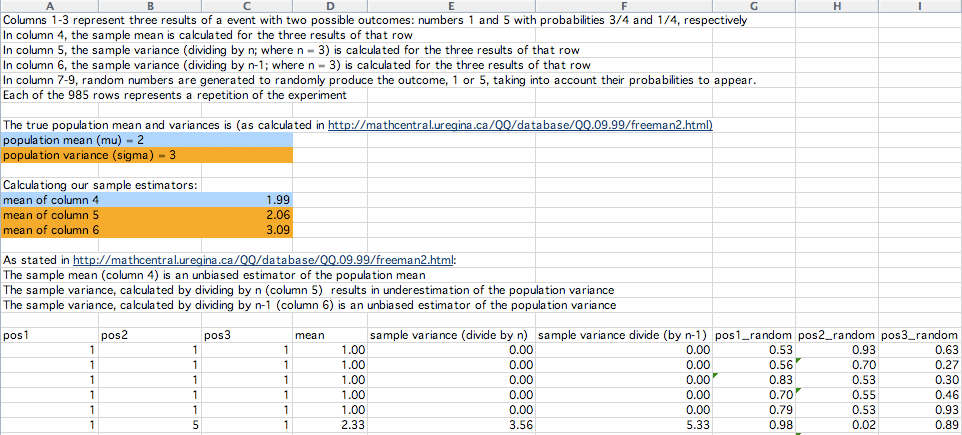A note on standard deviation

### Harley Weston, Department of Mathematics and Statistics, University of Regina

Suppose that you are in some course and have just received your grade on an exam. It is natural to ask how the rest of the class did on the exam so that you can put your grade in some context. Knowing the mean or median tells you the "center" or "middle" of the grades, but it would also be helpful to know some measure of the spread or variation in the grades.

Lets look at a small example. Suppose three classes of 5 students each write the same exam and the grades are:

Class 1Class 2Class 3
828267
788266
708266
584266
424265

Each of these classes has a mean,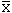, of 66 and yet there is great difference in the variation of the grades in each class. One measure of the variation is the range, which is the difference between the highest and lowest grades. In this example the range for the first two classes is 82 - 42 = 40 while the range for the third class is 67 - 65 = 2. The range is not a very good measure of variation here as classes 1 and 2 have the same range yet their variation seems to be quite different. One way to see this variation is to notice that in class 3 all the grades are very close to the mean, in class 1 some of the grades are close to the mean and some are far away and in class 2 all of the grades are a long way from the mean. It is this concept that leads to the definition of the standard deviation.

Lets look at class 1. For each student calculate the difference between the students grade and the mean.

Class 1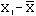8216
7812
704
58-8
42-24

The average of these differences could now be calculated as a measure of the variation, but this is zero. What is really needed is the distance from each grade to the mean not the difference. You could take the absolute value of each difference and then calculate the mean. This is called the mean deviation, i.e. mean deviation =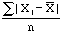, where n is the number of students in the class. For class 1 this is 64/5 = 12.8. Another way to deal with the negative differences is to square each difference before adding.

Class 1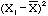8216256
7812144
70416
58-864
42-24576

The sum of this column is 1056. To find what is called the standard deviation, s, divide this sum by n-1 and then, since the sum is in square units, take the square root. For class 1 this gives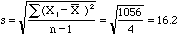A similar calculation gives a standard deviation of 21.9 for class 2 and 0.7 for class 3. So for class 3, where the grades are all close to the mean, the standard deviation is quite small, for class 1, where the grades are spread out between 42 and 82, the standard deviation is considerably larger and for class 2, where all the grades are far from the mean, the standard deviation is larger still. The standard deviation is the quantity most commonly used by statisticians to measure the variation in a data set.

The reason that the denominator in the calculation of s is n-1 deserves a comment. To look at this lets change the example. Suppose that I am interested in the number of hours per day that high school students in North America spend doing their mathematics homework. The "population" of interest is all high school students in North America, a very large number of people. Lets call this number N. My real interest is the mean and standard deviation of this population. When talking about a population statisticians usually use Greek letters to designate these quantities, so the mean of the population is written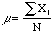, (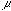is the Greek letter mu). Likewise the standard deviation is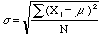, (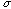is the Greek letter sigma). Notice that here the denomonator in the calculation is N.

Rather than trying to deal with this large population a statistician would usually select a "sample" of students, say n of them, and perform calculations on this smaller data set to estimate mu and sigma. Here n might be 25 or 30 or 100 or maybe even 1000, but certainly much smaller than N. To estimate mu it seems natural to use, the mean of the sample. Likewise to estimate sigma it seems reasonable to use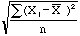, but this quantity tends to underestimate sigma, particularly for small n. For this and other technical reasons the quantity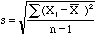is a usually preferred as the estimator to use for sigma.

If you have a calculator that computes the standard deviation it is a good exercise to see if it divides by n or n-1. Take the three number data set -1,0,1, calculate the standard deviation both ways by hand and then use your calculator to see which method it uses.

Footnote:

In the Spring of 2000 I received a request by a teacher to elaborate on the reasons why statisticians divide by n - 1 when calculating the sample variance. My reply was to describe an experiment that he could have his students perform in the classroom.

Harley

Footnote:

In December 2009 Javier Quílez Oliete, a doctoral student in Barcelona Spain, sent an Excel file where he simulated the experiment that I suggested in the previous footnote. He simulates repeatedly selecting a sample of size 3, with replacement, from a set with the two numbers 1 and 5 where the probability of selecting the 1 is 3/4 and the probability of selecting the 5 is 1/4. His simulation has 985 repetitions and for each of the 985 samples of size n = 3 he calculates the sample mean, the sample variance dividing by n, and the sample variance dividing by n - 1. He then compares these sample statistics to the population mean and variance.